Case Based Questions (MCQ)

Chapter 3 Class 12 Matrices
Serial order wise

## Question There are three families A, B and C. The number  of members in these families are given in the table  below.

 Men Women Children Family A 3 2 1 Family B 2 4 2 Family C 4 3 2

## The Daily expense of each man, woman and child are respectively Rs. 200, Rs. 100 and Rs. 50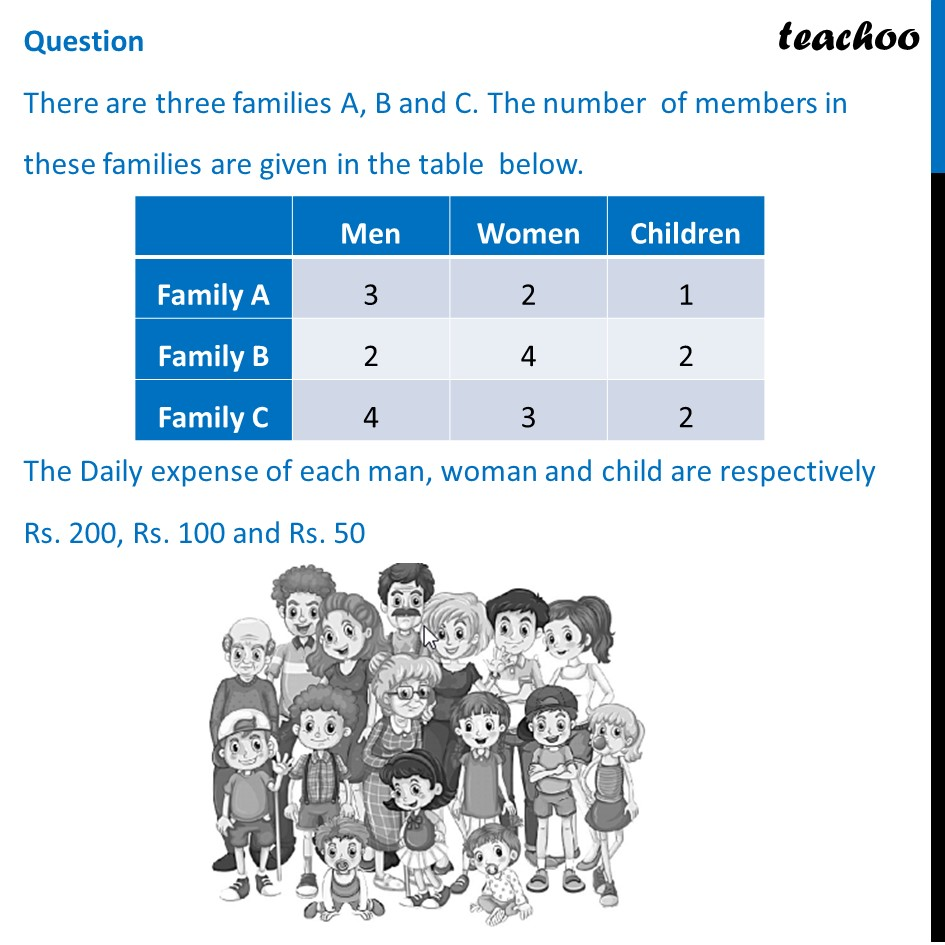## (d) 2950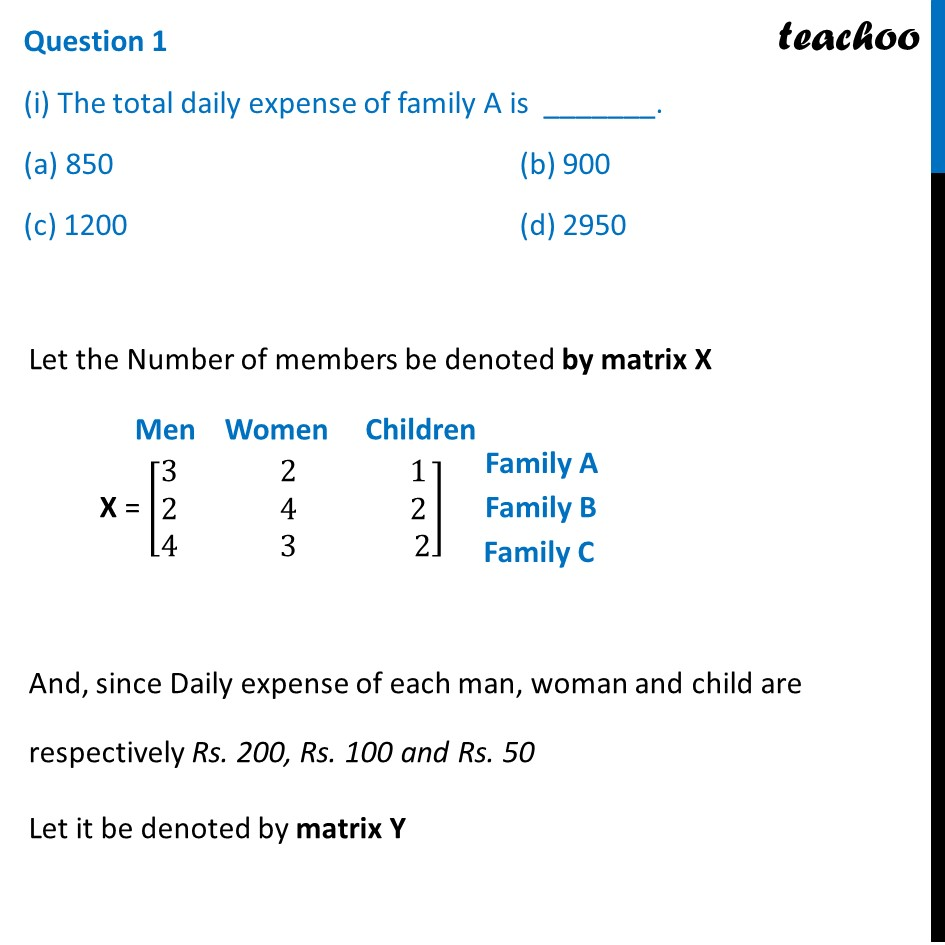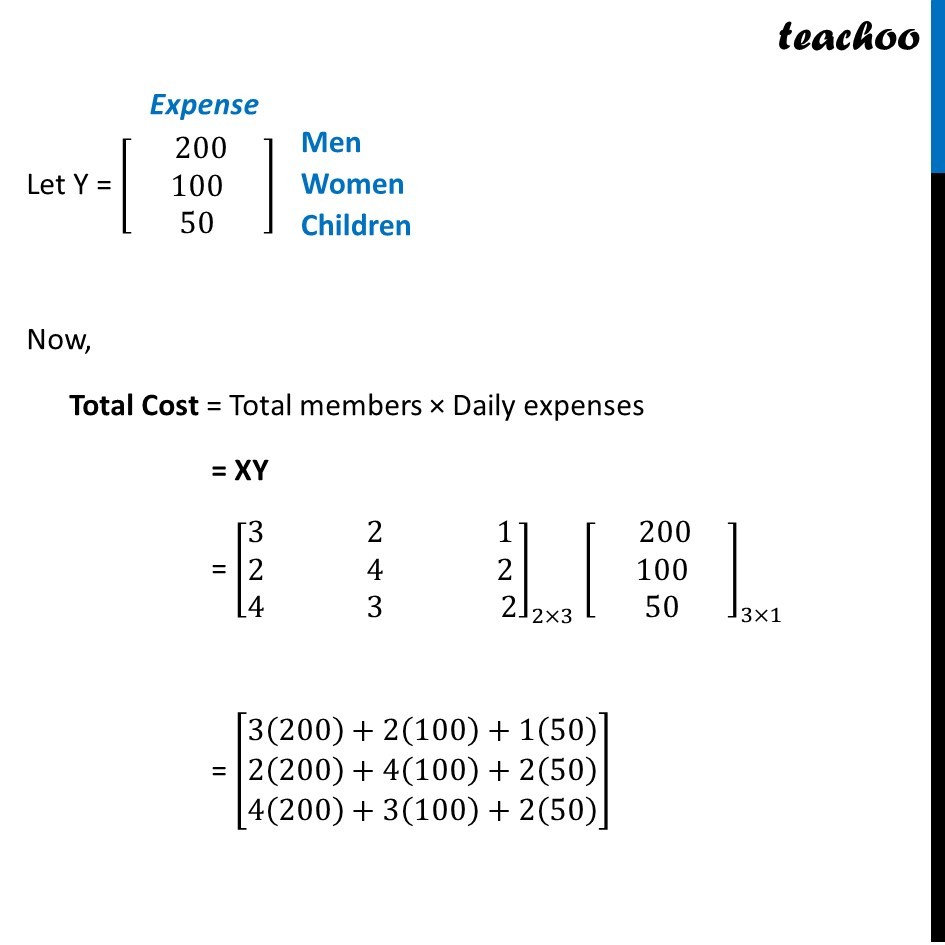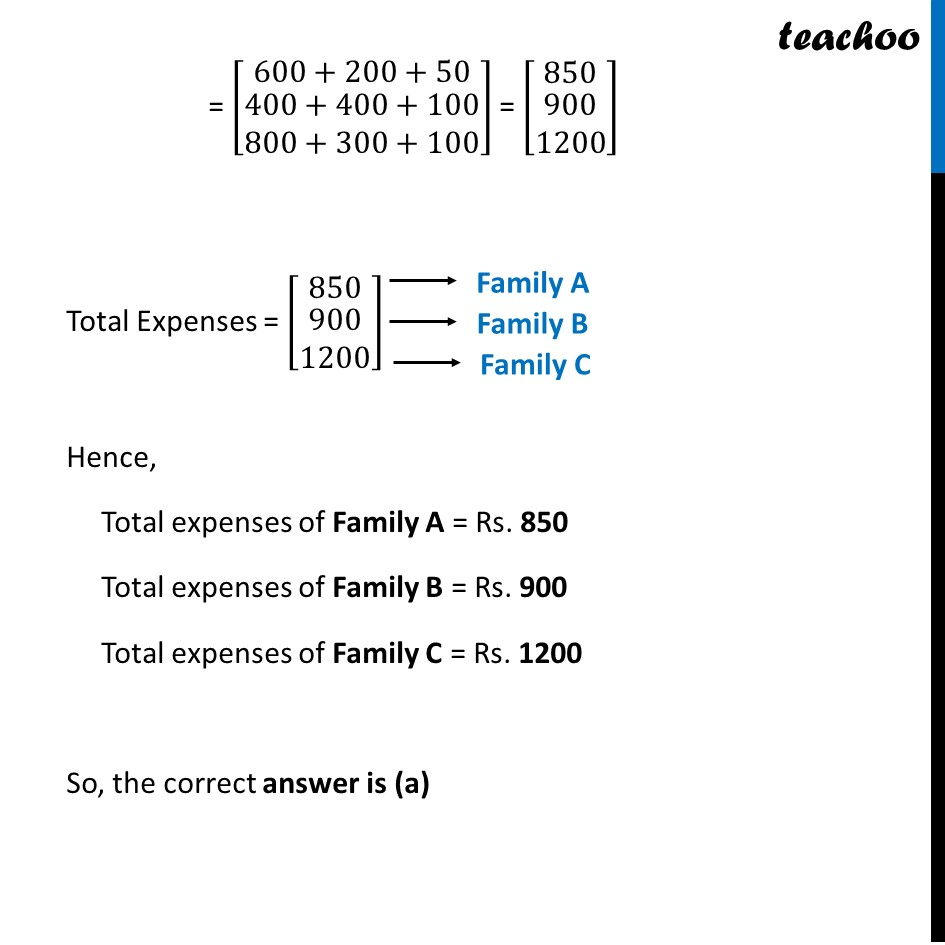## (d) 2950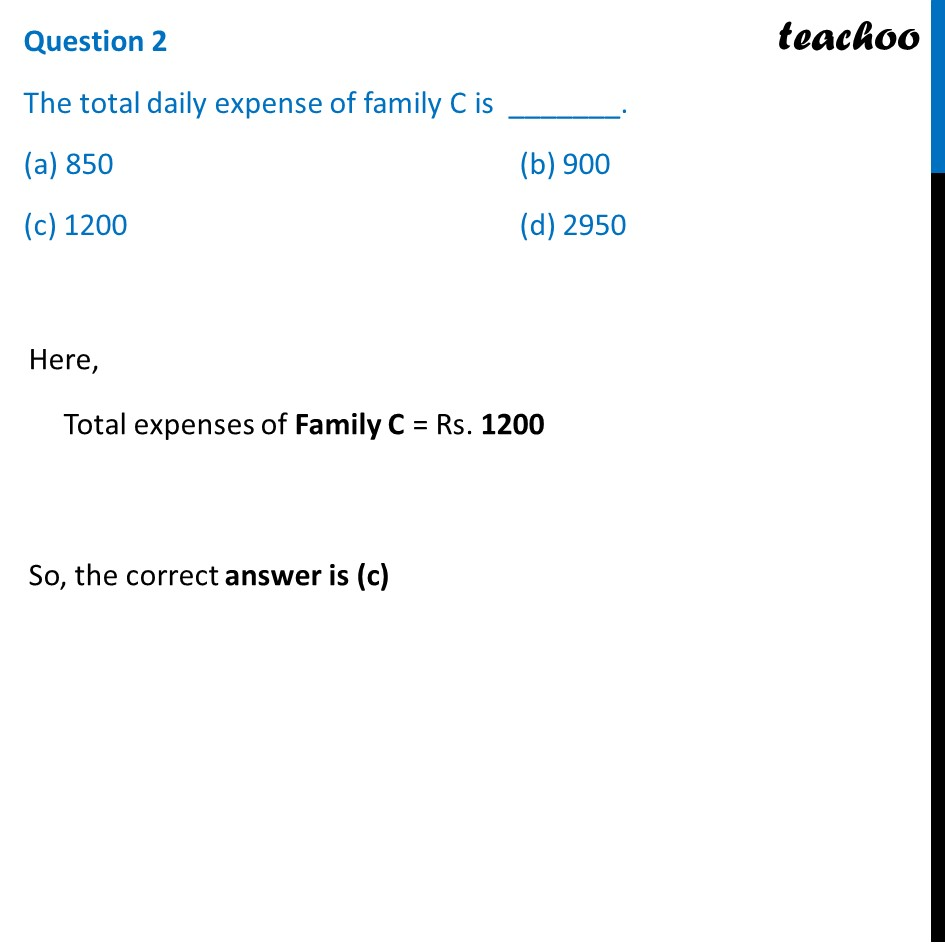## (d) 2950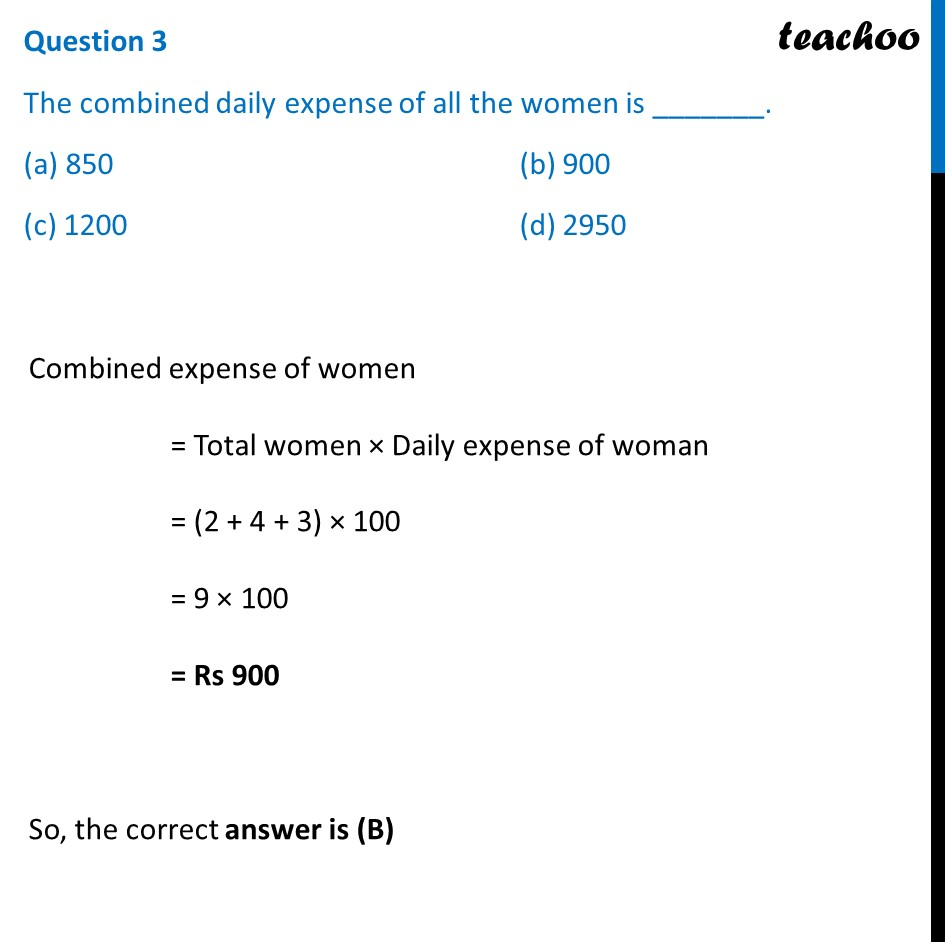## (d) D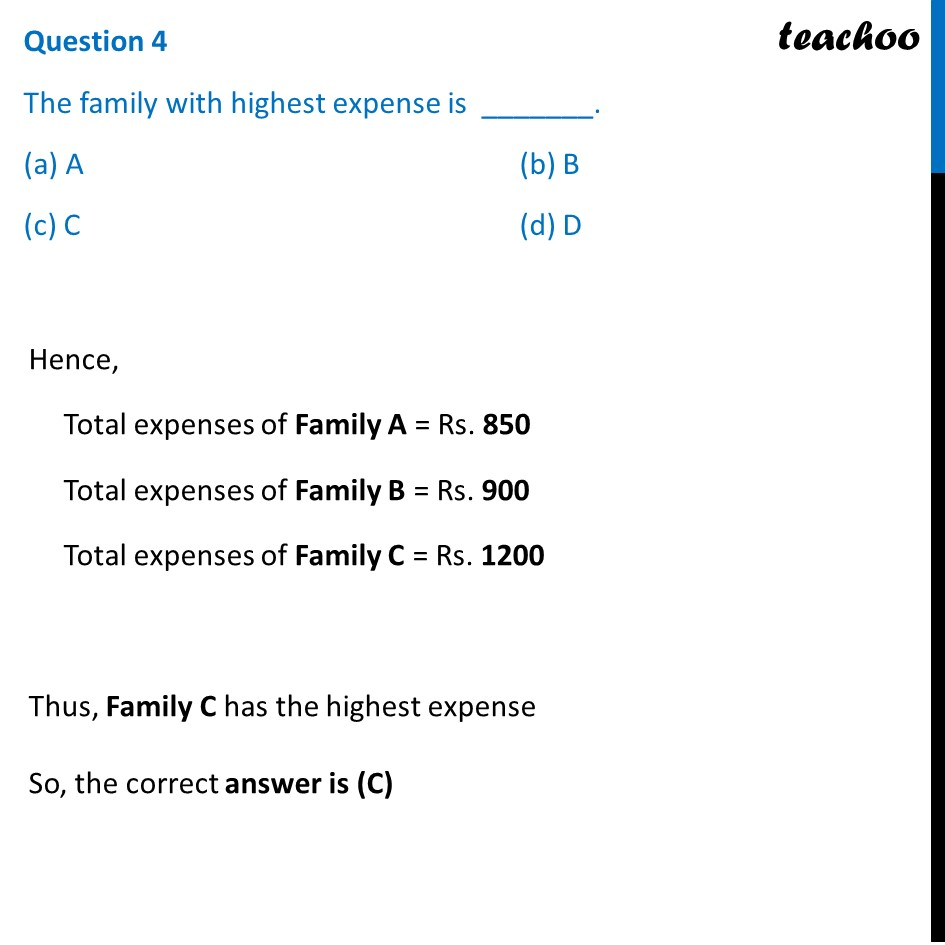## (d) 900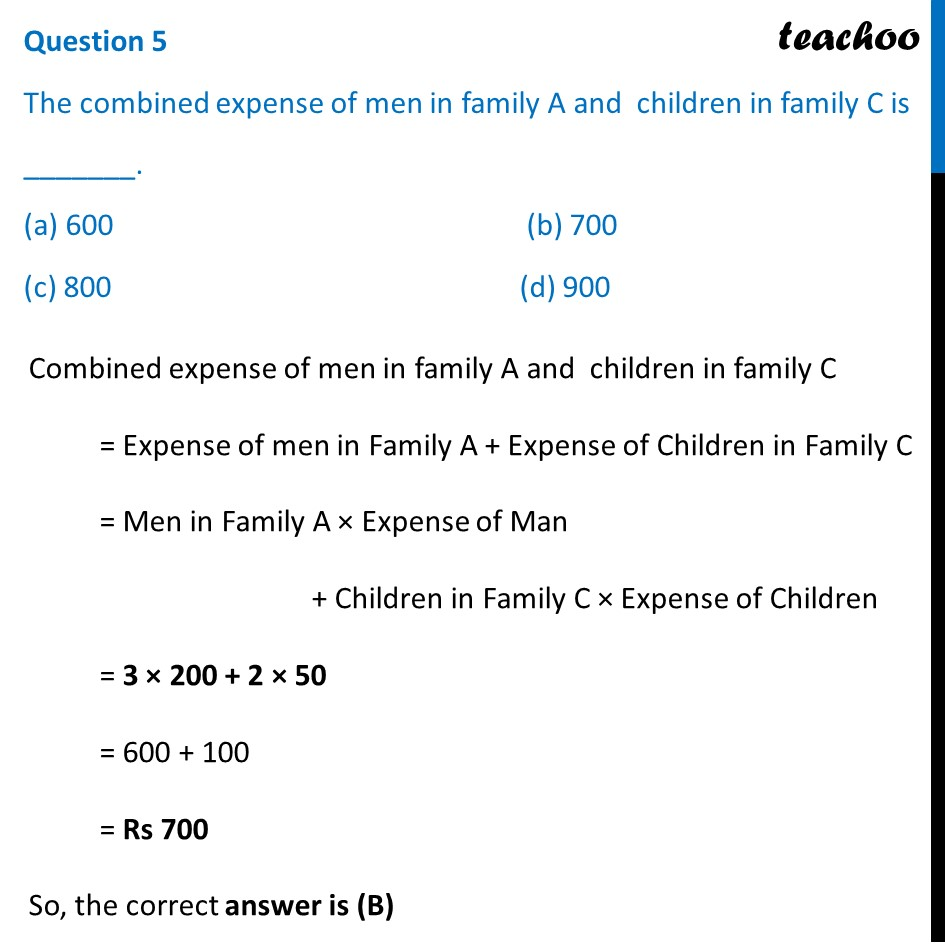Learn in your speed, with individual attention - Teachoo Maths 1-on-1 Class

### Transcript

Question There are three families A, B and C. The number of members in these families are given in the table below. The Daily expense of each man, woman and child are respectively Rs. 200, Rs. 100 and Rs. 50 Question 1 (i) The total daily expense of family A is _______. (a) 850 (b) 900 (c) 1200 (d) 2950 Let the Number of members be denoted by matrix X X = [■8(3& 2& 1@2& 4& 2@4& 3& 2)] And, since Daily expense of each man, woman and child are respectively Rs. 200, Rs. 100 and Rs. 50 Let it be denoted by matrix Y Let Y = [■8( 200 @100@50)] Now, Total Cost = Total members × Daily expenses = XY = [■8(3& 2& 1@2& 4& 2@4& 3& 2)]_(2×3) [■8( 200 @100@50)]_(3×1) = [■8(3(200)+2(100)+1(50)@█(2(200)+4(100)+2(50)@4(200)+3(100)+2(50) ))] = [■8(600+200+50@█(400+400+100@800+300+100))] = [■8(850@█(900@1200))] Total Expenses = [■8(850@█(900@1200))] Hence, Total expenses of Family A = Rs. 850 Total expenses of Family B = Rs. 900 Total expenses of Family C = Rs. 1200 So, the correct answer is (a) Question 2 The total daily expense of family C is _______. (a) 850 (b) 900 (c) 1200 (d) 2950 Here, Total expenses of Family C = Rs. 1200 So, the correct answer is (c) Question 3 The combined daily expense of all the women is _______. (a) 850 (b) 900 (c) 1200 (d) 2950 Combined expense of women = Total women × Daily expense of woman = (2 + 4 + 3) × 100 = 9 × 100 = Rs 900 So, the correct answer is (B) Question 4 The family with highest expense is _______. (a) A (b) B (c) C (d) D Hence, Total expenses of Family A = Rs. 850 Total expenses of Family B = Rs. 900 Total expenses of Family C = Rs. 1200 Thus, Family C has the highest expense So, the correct answer is (C) Question 5 The combined expense of men in family A and children in family C is _______. (a) 600 (b) 700 (c) 800 (d) 900 Combined expense of men in family A and children in family C = Expense of men in Family A + Expense of Children in Family C = Men in Family A × Expense of Man + Children in Family C × Expense of Children = 3 × 200 + 2 × 50 = 600 + 100 = Rs 700 So, the correct answer is (B)